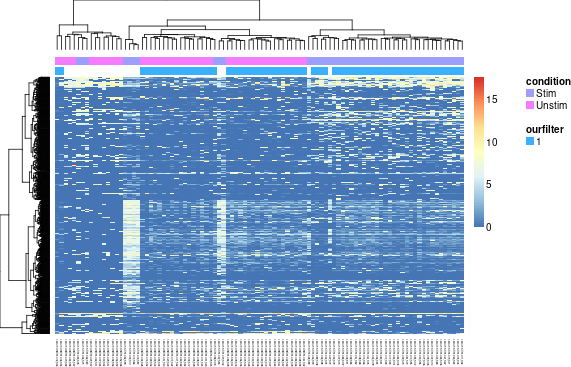# 1 Overview

We will learn how to use the package MAST to analyze single cell RNAseq experiments.

Starting from a matrix of counts of transcripts (cells by transcripts), we will discuss the preliminary steps of quality control, filtering, and exploratory data analysis. Once we are satisfied that we have high-quality expression, we will consider tests for differential expression and ways to visualize results. It is often helpful to synthesize from gene-level into module-level statements. Therefore, we will learn how to use MAST to test for gene set enrichment.

We will apply these methods to a data set of Mucosal Associated Invariant T cells (MAITs), measured on the Fluidigm C1. Half of the cells have been cytokine stimulated.

suppressPackageStartupMessages({
library(ggplot2)
library(GGally)
library(GSEABase)
library(limma)
library(reshape2)
library(data.table)
library(knitr)
library(TxDb.Hsapiens.UCSC.hg19.knownGene)
library(stringr)
library(NMF)
library(rsvd)
library(RColorBrewer)
library(MAST)
})
#options(mc.cores = detectCores() - 1) #if you have multiple cores to spin
options(mc.cores = 1)
knitr::opts_chunk$set(message = FALSE,error = FALSE,warning = FALSE,cache = FALSE,fig.width=8,fig.height=6) # 2 Loading and transforming data freq_expressed <- 0.2 FCTHRESHOLD <- log2(1.5) First, let’s set some constants that we can use throughout this analysis. data(maits, package='MAST') dim(maits$expressionmat)
head(maits$cdat) head(maits$fdat)

Next, let’s load the data, which consists of a matrix of log2 + 1 transcripts per million (TPM), as output by RSEM. Internally, we use the packages RNASeqPipelineR and preprocessData to facilitate aligning and quantitating the raw reads. This is an experiment on Mucosal Associated Invariant T-cells from a single healthy donor. A number of cells were subjected to reverse transcription and library prep immediately, while others were first stimulated for 24 hours with a cytokine cocktail.

scaRaw <- FromMatrix(t(maits$expressionmat), maits$cdat, maits$fdat) FromMatrix constructs an object from a matrix (genes $$\times$$ cells) of expression, a data.frame of cell-level covariance and a data.frame of feature-level covariates. In this case, there are 96 single cells measured over 16302 genes. We derive from the SummarizedExperiment class, which makes it easy for feature (row) and cell (column) metadata to come along for the ride. There is also a constructor, FromFlatDF for flattened data where measurements, and cell and feature covariates are intermingled. ## 2.1 Exploratory Data Analysis Let’s explore these data with a heatmap and some PCA. aheatmap(assay(scaRaw[1:1000,]), labRow='', annCol=as.data.frame(colData(scaRaw)[,c('condition', 'ourfilter')]), distfun='spearman')set.seed(123) plotPCA <- function(sca_obj){ projection <- rpca(t(assay(sca_obj)), retx=TRUE, k=4)$x
colnames(projection)=c("PC1","PC2","PC3","PC4")
pca <- data.table(projection,  as.data.frame(colData(sca_obj)))
print(ggpairs(pca, columns=c('PC1', 'PC2', 'PC3', 'libSize', 'PercentToHuman', 'nGeneOn', 'exonRate'),
mapping=aes(color=condition), upper=list(continuous='blank')))
invisible(pca)
}

plotPCA(scaRaw)